﻿ 不完全CI下Wolbachia传播的离散竞争模型 Modeling Wolbachia Propagation underIncomplete Cytoplasmic Incompatibility by Discrete Competition Model

Vol. 09  No. 02 ( 2020 ), Article ID: 34189 , 13 pages
10.12677/AAM.2020.92018

Modeling Wolbachia Propagation under Incomplete Cytoplasmic Incompatibility by Discrete Competition Model

Yijie Li, Zhiming Guo*

School of Mathematics and Information Science, Guangzhou University, Guangzhou Guangdong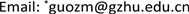Received: Jan. 25th, 2020; accepted: Feb. 7th, 2020; published: Feb. 14th, 2020ABSTRACT

Dengue fever is one of the most serious mosquito-borne infectious diseases. Using Wolbachia infection mosquitoes to control those diseases is an effective strategy. In this paper, a discrete competition model is established to study the dynamic of Wolbachia propagation under incomplete cytoplasmic incompatibility (CI). We systematically analyze the existing conditions of the equilibrium and global asymptotic behaviors of solutions to this model, then we give the conditions for successful diffusion and the influence of CI strength on the Wolbachia diffusion. Finally, we verify our findings by numerical simulations.

Keywords:Wolbachia, Incomplete CI, Competition, Discrete Model, Stability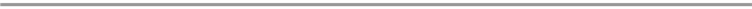1. 引言

2. 模型的建立及其分析

$\left\{\begin{array}{l}{x}_{t+1}={b}_{1}\frac{1}{1+{c}_{11}{x}_{t}+{c}_{12}{y}_{t}}{x}_{t},\\ {y}_{t+1}={b}_{2}\frac{1}{1+{c}_{21}{x}_{t}+{c}_{22}{y}_{t}}{y}_{t}.\end{array}$ (1)

$\left\{\begin{array}{l}{x}_{t+1}=\frac{{b}_{1}{x}_{t}}{1+\alpha \left({x}_{t}+{y}_{t}\right)}+\left(1-{d}_{1}\right){x}_{t},\\ {y}_{t+1}=\frac{{b}_{2}{y}_{t}}{1+\alpha \left({x}_{t}+{y}_{t}\right)}\left(1-q\frac{{x}_{t}}{{x}_{t}+{y}_{t}}\right)+\left(1-{d}_{2}\right){y}_{t}.\end{array}$ (2)

$\begin{array}{l}{x}_{t+1}\le \frac{{b}_{1}}{\alpha }+\left(1-{d}_{1}\right){x}_{t},\\ {y}_{t+1}\le \frac{{b}_{2}}{\alpha }+\left(1-{d}_{2}\right){y}_{t},\end{array}$

$\underset{t\to \infty }{\mathrm{lim}\mathrm{sup}}{x}_{t}\le \frac{{b}_{1}}{\alpha {d}_{1}},\text{ }\text{ }\text{ }\text{ }\text{ }\text{ }\text{ }\text{ }\underset{t\to \infty }{\mathrm{lim}\mathrm{sup}}{y}_{t}\le \frac{{b}_{2}}{\alpha {d}_{2}}.$

$\Gamma =\left\{\left(x,y\right)\in {R}^{2}|0\le x\le \frac{{b}_{1}}{\alpha {d}_{1}},0\le y\le \frac{{b}_{2}}{\alpha {d}_{2}}\right\}.$

$f\left(x,y\right)=\left\{\begin{array}{l}\frac{{b}_{2}y}{1+\alpha \left(x+y\right)}\left(1-q\frac{x}{x+y}\right)+\left(1-{d}_{2}\right)y,\text{ }\text{ }\text{ }\text{ }\text{ }\text{ }\text{ }\text{ }\text{ }\text{ }\text{ }\left(x,y\right)\ne \left(0,0\right),\\ 0\text{ },\text{ }\text{ }\text{ }\text{ }\text{ }\text{ }\text{ }\text{ }\text{ }\text{ }\text{ }\text{ }\text{ }\text{ }\text{ }\text{ }\text{ }\text{ }\text{ }\text{ }\text{ }\text{ }\text{ }\text{ }\text{ }\text{ }\text{ }\text{ }\text{ }\text{ }\text{ }\text{ }\text{ }\text{ }\text{ }\text{ }\text{ }\text{ }\text{ }\text{ }\text{ }\text{ }\text{ }\text{ }\text{ }\text{ }\text{ }\text{ }\text{ }\text{ }\text{ }\text{ }\text{ }\text{ }\text{ }\text{ }\text{ }\text{ }\text{ }\text{ }\text{ }\text{ }\text{ }\text{ }\text{ }\text{ }\text{ }\text{ }\text{ }\text{ }\text{ }\text{ }\text{ }\text{ }\text{ }\text{ }\text{ }\text{ }\text{ }\text{ }\text{ }\text{ }\text{ }\text{ }\text{ }\text{ }\text{ }\text{ }\text{ }\text{ }\text{ }\text{ }\text{ }\text{ }\text{ }\text{ }\text{ }\text{ }\text{ }\text{ }\text{ }\text{ }\text{ }\text{ }\text{ }\text{ }\text{ }\text{ }\text{ }\text{ }\text{ }\text{ }\text{ }\text{ }\text{ }\text{ }\text{ }\text{ }\text{ }\text{ }\text{ }\text{ }\text{ }\text{ }\text{ }\text{ }\text{ }\text{ }\text{ }\text{ }\text{ }\text{ }\text{ }\text{ }\text{ }\text{ }\text{ }\text{ }\text{ }\text{ }\text{ }\text{ }\text{ }\text{ }\text{ }\text{ }\text{ }\text{ }\text{ }\text{ }\text{ }\text{ }\text{ }\text{ }\text{ }\text{ }\left(x,y\right)=\left(0,0\right).\end{array}$

$\left\{\begin{array}{l}{x}_{t+1}=\frac{{b}_{1}{x}_{t}}{1+\alpha \left({x}_{t}+{y}_{t}\right)}+\left(1-{d}_{1}\right){x}_{t},\\ {y}_{t+1}=f\left({x}_{t},{y}_{t}\right).\end{array}$

$\left\{\begin{array}{l}x=\frac{{b}_{1}x}{1+\alpha \left(x+y\right)}+\left(1-{d}_{1}\right)x,\\ y=\frac{{b}_{2}y}{1+\alpha \left(x+y\right)}\left(1-q\frac{x}{x+y}\right)+\left(1-{d}_{2}\right)y.\end{array}$ (3)

${E}_{3}\left(\frac{1}{\alpha q}\left(\frac{{b}_{1}}{{d}_{1}}-1\right)\left(1-\frac{{b}_{1}{d}_{2}}{{b}_{2}{d}_{1}}\right),\frac{1}{\alpha }\left(\frac{{b}_{1}}{{d}_{1}}-1\right)\left[1-\frac{1}{q}\left(1-\frac{{b}_{1}{d}_{2}}{{b}_{2}{d}_{1}}\right)\right]\right)$

a) 若 ${b}_{1}\le {d}_{1},{b}_{2}\le {d}_{2}$，则 ${E}_{0}$ 是全局渐近稳定的；否则， ${E}_{0}$ 是不稳定的。

b) 若 ${b}_{1}>{d}_{1}$，则当 $\frac{{b}_{1}}{{d}_{1}}>\frac{{b}_{2}}{{d}_{2}}\left(1-q\right)$ 时， ${E}_{1}$ 是局部渐近稳定的；当 $\frac{{b}_{1}}{{d}_{1}}<\frac{{b}_{2}}{{d}_{2}}\left(1-q\right)$ 时， ${E}_{1}$ 是一个鞍点。进一步，若 ${E}_{1}$ 是局部渐近稳定的且 ${b}_{2}\le {d}_{2}$，则 ${E}_{1}$ 是全局渐近稳定的。

c) 若 ${b}_{2}>{d}_{2}$，则当 $\frac{{b}_{2}}{{d}_{2}}>\frac{{b}_{1}}{{d}_{1}}$ 时， ${E}_{2}$ 是局部渐近稳定的；当 $\frac{{b}_{2}}{{d}_{2}}<\frac{{b}_{1}}{{d}_{1}}$ 时， ${E}_{2}$ 是一个鞍点。进一步，若 ${E}_{2}$ 是局部渐近稳定的且 ${b}_{1}\le {d}_{1}$，则 ${E}_{2}$ 是全局渐近稳定的。

$J=\left(\begin{array}{cc}\frac{{b}_{1}\left(1+\alpha y\right)}{{\left[1+\alpha \left(x+y\right)\right]}^{2}}+1-{d}_{1}& \frac{-\alpha {b}_{1}x}{{\left[1+\alpha \left(x+y\right)\right]}^{2}}\\ \frac{q{b}_{2}y\left[\alpha \left({x}^{2}-{y}^{2}\right)-y\right]-\alpha {b}_{2}y{\left(x+y\right)}^{2}}{{\left[1+\alpha \left(x+y\right)\right]}^{2}{\left(x+y\right)}^{2}}& \frac{q{b}_{2}x\left[\alpha \left({y}^{2}-{x}^{2}\right)-x\right]+{b}_{2}\left(1+\alpha x\right){\left(x+y\right)}^{2}}{{\left[1+\alpha \left(x+y\right)\right]}^{2}{\left(x+y\right)}^{2}}+1-{d}_{2}\end{array}\right).$ (4)

${J}_{1}={\left(\begin{array}{cc}\frac{{d}_{1}^{2}}{{b}_{1}}+1-{d}_{1}& \frac{{d}_{1}^{2}}{{b}_{1}}-{d}_{1}\\ 0& \frac{{b}_{2}{d}_{1}\left(1-q\right)}{{b}_{1}}+1-{d}_{2}\end{array}\right)}_{,}$ (5)

${J}_{2}={\left(\begin{array}{cc}\frac{{b}_{1}{d}_{2}}{{b}_{2}}+1-{d}_{1}& 0\\ \frac{{d}_{2}^{2}}{{b}_{2}}-{d}_{2}\left(1+q\right)& \frac{{d}_{2}^{2}}{{b}_{2}}+1-{d}_{2}\end{array}\right)}_{,}$ (6)

a) 若 ${b}_{1}\le {d}_{1},{b}_{2}\le {d}_{2}$，则系统只有一个平凡平衡点 ${E}_{0}$。当 ${b}_{1}<{d}_{1},{b}_{2}<{d}_{2}$ 时，由模型(2)可得

$\begin{array}{l}0\le {x}_{t+1}\le \left({b}_{1}-{d}_{1}+1\right){x}_{t},\\ 0\le {y}_{t+1}\le \left({b}_{2}-{d}_{2}+1\right){y}_{t},\end{array}$

${x}_{t+1}=\left[\frac{{b}_{1}}{1+\alpha \left({x}_{t}+{y}_{t}\right)}+1-{d}_{1}\right]{x}_{t},$

${y}_{t+1}=\left[\frac{{b}_{2}}{1+\alpha \left({x}_{t}+{y}_{t}\right)}\left(1-q\frac{{x}_{t}}{{x}_{t}+{y}_{t}}\right)+1-{d}_{2}\right]{y}_{t},$

${x}_{t+1}=\left(\frac{{b}_{1}}{1+\alpha {x}_{t}}+1-{d}_{1}\right){x}_{t},$

b) ${b}_{1}>{d}_{1}$，则当 $\frac{{b}_{1}}{{d}_{1}}>\frac{{b}_{2}}{{d}_{2}}\left(1-q\right)$ 时，由 ${J}_{1}$ 的所有特征值的绝对值都小于1可知 ${E}_{1}$ 是局部渐近稳定的；而当 $\frac{{b}_{1}}{{d}_{1}}<\frac{{b}_{2}}{{d}_{2}}\left(1-q\right)$ 时，由 ${J}_{1}$ 的一个特征值的绝对值小于1，另一个特征值大于1，可知 ${E}_{1}$ 是一个鞍点。进一步，若 ${E}_{1}$ 是局部渐近稳定的且 ${b}_{2}\le {d}_{2}$，模型只存在两个平衡点 ${E}_{0}$${E}_{1}$，由a)知此时 ${E}_{0}$ 不稳定，则根据文献  中的定理，所有的有界轨线将最终趋于一个平衡点，因此所有轨线只能趋于 ${E}_{1}$，可得 ${E}_{1}$ 是全局渐近稳定的。

c) 这里的证明与b)类似，所以省略。

$y=-x+\frac{1}{\alpha }\left(\frac{{b}_{1}}{{d}_{1}}-1\right),$

${L}_{1}$ 表示，这是一条斜率为−1，截距为 $\frac{1}{\alpha }\left(\frac{{b}_{1}}{{d}_{1}}-1\right)$ 的直线段。由模型(2)的第二个式子可得水平等倾线

$\alpha {x}^{2}+2\alpha xy+\alpha {y}^{2}+\left[1+\frac{{b}_{2}}{{d}_{2}}\left(q-1\right)\right]x+\left(1-\frac{{b}_{2}}{{d}_{2}}\right)y=0,$

${L}_{2}$ 表示，这是经过三个不动点 $\left(0,0\right),\left(0,\frac{1}{\alpha }\left(\frac{{b}_{2}}{{d}_{2}}-1\right)\right),\left(\frac{1}{\alpha }\left[\frac{{b}_{2}}{{d}_{2}}\left(1-q\right)-1\right],0\right)$ 的抛物线，见图1Figure 1. The three possible cases of L1and L2

Case A的条件为

${b}_{1}>{d}_{1},\text{ }\text{ }\text{ }\text{ }\text{ }\text{ }\text{ }{b}_{2}>{d}_{2},\text{ }\text{ }\text{ }\text{ }\text{ }\text{ }\text{ }\frac{{b}_{1}}{{d}_{1}}>\frac{{b}_{2}}{{d}_{2}}.$

Case B的条件为

${b}_{1}>{d}_{1},\text{ }\text{ }\text{ }\text{ }\text{ }\text{ }\text{ }{b}_{2}>{d}_{2},\text{ }\text{ }\text{ }\text{ }\text{ }\text{ }\text{ }\frac{{b}_{2}}{{d}_{2}}>\frac{{b}_{1}}{{d}_{1}}>\frac{{b}_{2}}{{d}_{2}}\left(1-q\right).$

Case C的条件为

${b}_{1}>{d}_{1},\text{ }\text{ }\text{ }\text{ }\text{ }\text{ }\text{ }{b}_{2}>{d}_{2},\text{ }\text{ }\text{ }\text{ }\text{ }\text{ }\text{ }\frac{{b}_{1}}{{d}_{1}}<\frac{{b}_{2}}{{d}_{2}}\left(1-q\right).$

a) 若 $\frac{{b}_{1}}{{d}_{1}}>\frac{{b}_{2}}{{d}_{2}}$，则 ${E}_{1}$ 是全局渐近稳定的， ${E}_{2}$ 是一个鞍点。

b) 若 $\frac{{b}_{2}}{{d}_{2}}>\frac{{b}_{1}}{{d}_{1}}>\frac{{b}_{2}}{{d}_{2}}\left(1-q\right)$，则 ${E}_{1}$${E}_{2}$ 都是局部渐近稳定的， ${E}_{3}$ 是一个鞍点。

c) 若 $\frac{{b}_{1}}{{d}_{1}}<\frac{{b}_{2}}{{d}_{2}}\left(1-q\right)$，则 ${E}_{2}$ 是全局渐近稳定的， ${E}_{1}$ 是一个鞍点。

b) 在此条件下(即Case B)，由 ${J}_{1}$${J}_{2}$ 可得 ${E}_{1}$${E}_{2}$ 的所有特征值的绝对值都小于1，所以 ${E}_{1}$${E}_{2}$ 都是局部渐近稳定的。下面讨论 ${E}_{3}$ 的稳定性，为了表达方便，记

${E}_{3}=\left({x}^{*},{y}^{*}\right)=\left(\frac{1}{\alpha q}\left(\frac{{b}_{1}}{{d}_{1}}-1\right)\left(1-\frac{{b}_{1}{d}_{2}}{{b}_{2}{d}_{1}}\right),\frac{1}{\alpha }\left(\frac{{b}_{1}}{{d}_{1}}-1\right)\left[1-\frac{1}{q}\left(1-\frac{{b}_{1}{d}_{2}}{{b}_{2}{d}_{1}}\right)\right]\right).$

${J}_{3}={\left(\begin{array}{cc}\frac{{d}_{1}^{2}\left(1+\alpha {y}^{*}\right)}{{b}_{1}}+1-{d}_{1}& -\frac{\alpha {d}_{1}^{2}{x}^{*}}{{b}_{1}}\\ \frac{q{b}_{2}{y}^{*}\left[\left(\frac{{b}_{1}}{{d}_{1}}-1\right){x}^{*}-\frac{{b}_{1}}{{d}_{1}}{y}^{*}\right]-\alpha {b}_{2}{y}^{*}{\left({x}^{*}+{y}^{*}\right)}^{2}}{{\left[\frac{{b}_{1}}{{d}_{1}}\left({x}^{*}+{y}^{*}\right)\right]}^{2}}& \frac{q{b}_{2}{x}^{*}\left[\left(\frac{{b}_{1}}{{d}_{1}}-1\right){y}^{*}-\frac{{b}_{1}}{{d}_{1}}{x}^{*}\right]+{b}_{2}\left(1+\alpha {x}^{*}\right){\left({x}^{*}+{y}^{*}\right)}^{2}}{{\left[\frac{{b}_{1}}{{d}_{1}}\left({x}^{*}+{y}^{*}\right)\right]}^{2}}+1-{d}_{2}\end{array}\right)}_{·}$

(7)

$|tr{J}_{3}|<1+\mathrm{det}{J}_{3}<2$

$\left(1\right).\text{}\text{ }\text{ }\text{ }1+\mathrm{det}{J}_{3}<2;\text{}\left(2\right)\text{ }\text{.}\text{ }\text{ }-1-\mathrm{det}{J}_{3}

$\begin{array}{l}\mathrm{det}{J}_{3}=\left(\frac{{d}_{1}^{2}}{{b}_{1}}+1-{d}_{1}\right)\frac{q{b}_{2}{x}^{*}\left[\left(\frac{{b}_{1}}{{d}_{1}}-1\right){y}^{*}-\frac{{b}_{1}}{{d}_{1}}{x}^{*}\right]+{b}_{2}\left(1+\alpha {x}^{*}\right){\left({x}^{*}+{y}^{*}\right)}^{2}}{{\left[\frac{{b}_{1}}{{d}_{1}}\left({x}^{*}+{y}^{*}\right)\right]}^{2}}\\ \text{ }\text{ }\text{ }\text{ }\text{ }\text{ }\text{ }\text{ }\text{ }\text{ }\text{ }\text{ }\text{ }\text{ }\text{ }\text{ }\text{ }\text{ }\text{ }\text{ }\text{ }\text{ }\text{ }\text{ }\text{ }\text{ }\text{ }\text{ }\text{ }\text{ }\text{ }\text{ }\text{ }\text{ }+\left(1-{d}_{2}\right)\frac{{d}_{1}^{2}\left(1+\alpha {y}^{*}\right)}{{b}_{1}}+\frac{{d}_{1}^{4}\left[\alpha {b}_{2}{y}^{*}\left({x}^{*}+{y}^{*}\right)-\alpha q{b}_{2}{x}^{*}{y}^{*}\right]}{{b}_{1}^{3}\left({x}^{*}+{y}^{*}\right)}+\left(1-{d}_{1}\right)\left(1-{d}_{2}\right),\end{array}$

$tr{J}_{3}=\frac{q{b}_{2}{x}^{*}\left[\left(\frac{{b}_{1}}{{d}_{1}}-1\right){y}^{*}-\frac{{b}_{1}}{{d}_{1}}{x}^{*}\right]+{b}_{2}\left(1+\alpha {x}^{*}\right){\left({x}^{*}+{y}^{*}\right)}^{2}}{{\left[\frac{{b}_{1}}{{d}_{1}}\left({x}^{*}+{y}^{*}\right)\right]}^{2}}+\frac{{d}_{1}^{2}\left(1+\alpha {y}^{*}\right)}{{b}_{1}}+2-{d}_{1}-{d}_{2}.$

$\begin{array}{l}\left(\frac{{d}_{1}^{2}}{{b}_{1}}-{d}_{1}\right)\frac{q{b}_{2}{x}^{*}\left[\left(\frac{{b}_{1}}{{d}_{1}}-1\right){y}^{*}-\frac{{b}_{1}}{{d}_{1}}{x}^{*}\right]+{b}_{2}\left(1+\alpha {x}^{*}\right){\left({x}^{*}+{y}^{*}\right)}^{2}}{{\left[\frac{{b}_{1}}{{d}_{1}}\left({x}^{*}+{y}^{*}\right)\right]}^{2}}\\ -\frac{{d}_{1}^{2}{d}_{2}\left(1+\alpha {y}^{*}\right)}{{b}_{1}}+\frac{{d}_{1}^{4}\left[\alpha {b}_{2}{y}^{*}\left({x}^{*}+{y}^{*}\right)-\alpha q{b}_{2}{x}^{*}{y}^{*}\right]}{{b}_{1}^{3}\left({x}^{*}+{y}^{*}\right)}+{d}_{1}{d}_{2}>0.\end{array}$

$\begin{array}{l}\frac{q{b}_{2}\left(\frac{{d}_{1}}{{b}_{1}}-1\right){x}^{*}\left[\left(\frac{{b}_{1}}{{d}_{1}}-1\right){y}^{*}-\frac{{b}_{1}}{{d}_{1}}{x}^{*}\right]}{{\left[\frac{{b}_{1}}{{d}_{1}}\left({x}^{*}+{y}^{*}\right)\right]}^{2}}+\frac{{d}_{1}^{2}{b}_{2}}{{b}_{1}{}^{2}}\left(\frac{{d}_{1}}{{b}_{1}}-1\right)\left(1+\alpha {x}^{*}\right)\\ +{d}_{2}\left[1-\frac{{d}_{1}}{{b}_{1}}\left(1+\alpha {y}^{*}\right)\right]+\frac{{d}_{1}^{3}\left[\alpha {b}_{2}{y}^{*}\left({x}^{*}+{y}^{*}\right)-\alpha q{b}_{2}{x}^{*}{y}^{*}\right]}{{b}_{1}^{3}\left({x}^{*}+{y}^{*}\right)}>0,\end{array}$

${E}_{3}=\left({x}^{*},{y}^{*}\right)$ 代入上式，有

$\begin{array}{l}\frac{{d}_{1}^{2}{b}_{2}}{{b}_{1}^{2}}\left(\frac{{d}_{1}}{{b}_{1}}-1\right)\left(1-\frac{{b}_{1}{d}_{2}}{{b}_{2}{d}_{1}}\right)\left[\frac{{b}_{1}}{{d}_{1}}-1-\frac{1}{q}\left(2\frac{{b}_{1}}{{d}_{1}}-1\right)\left(1-\frac{{b}_{1}{d}_{2}}{{b}_{2}{d}_{1}}\right)\right]+\frac{{d}_{1}^{2}{b}_{2}}{{b}_{1}^{2}}\left(\frac{{b}_{1}}{{d}_{1}}-1\right)\left[1-\frac{1}{q}\left(1-\frac{{b}_{1}{d}_{2}}{{b}_{2}{d}_{1}}\right)\right]\\ +\frac{{d}_{1}^{2}{b}_{2}}{{b}_{1}^{2}}\left(\frac{{d}_{1}}{{b}_{1}}-1\right)\left[1+\frac{1}{q}\left(\frac{{b}_{1}}{{d}_{1}}-1\right)\left(1-\frac{{b}_{1}{d}_{2}}{{b}_{2}{d}_{1}}\right)\right]+{d}_{2}\left(1-\frac{{d}_{1}}{{b}_{1}}\left(1+\left(\frac{{b}_{1}}{{d}_{1}}-1\right)\left[1-\frac{1}{q}\left(1-\frac{{b}_{1}{d}_{2}}{{b}_{2}{d}_{1}}\right)\right]\right)\right)\\ =\frac{{d}_{1}{b}_{2}}{{b}_{1}}\left(\frac{{d}_{1}}{{b}_{1}}-1\right)\left(\left(1-\frac{{b}_{1}{d}_{2}}{{b}_{2}{d}_{1}}\right)\left[1-\frac{1}{q}\left(1-\frac{{b}_{1}{d}_{2}}{{b}_{2}{d}_{1}}\right)\right]+\frac{{d}_{2}}{{b}_{2}}\left[1+\frac{1}{q}\left(\frac{{b}_{1}}{{d}_{1}}-1\right)\left(1-\frac{{b}_{1}{d}_{2}}{{b}_{2}{d}_{1}}\right)\right]\right)+{d}_{2}\end{array}$

$\begin{array}{l}-\frac{{d}_{1}{d}_{2}}{{b}_{1}}\left(1+\left(\frac{{b}_{1}}{{d}_{1}}-1\right)\left[1-\frac{1}{q}\left(1-\frac{{b}_{1}{d}_{2}}{{b}_{2}{d}_{1}}\right)\right]\right)+\frac{{d}_{1}^{2}{d}_{2}}{{b}_{1}{}^{2}}\left(\frac{{b}_{1}}{{d}_{1}}-1\right)\left[1-\frac{1}{q}\left(1-\frac{{b}_{1}{d}_{2}}{{b}_{2}{d}_{1}}\right)\right]\\ =\left[\frac{{d}_{1}{b}_{2}}{{b}_{1}}\left(\frac{{d}_{1}}{{b}_{1}}-1\right)+\frac{{d}_{1}{d}_{2}}{{b}_{1}}\left(1-\frac{{d}_{1}}{{b}_{1}}\right)\right]\left[1-\frac{1}{q}\left(1-\frac{{b}_{1}{d}_{2}}{{b}_{2}{d}_{1}}\right)\right]+\frac{{d}_{1}{d}_{2}}{{b}_{1}}\left(\frac{{d}_{1}}{{b}_{1}}-1\right)\\ \text{ }\text{ }\text{ }\text{ }\text{ }\text{ }\text{ }\text{ }+{d}_{2}\left(1-\frac{{d}_{1}}{{b}_{1}}\right)+\frac{{d}_{1}{d}_{2}}{q{b}_{1}}\left(\frac{{d}_{1}}{{b}_{1}}-1\right)\left(\frac{{b}_{1}}{{d}_{1}}-1\right)\left(1-\frac{{b}_{1}{d}_{2}}{{b}_{2}{d}_{1}}\right)\\ =\frac{1}{q}\left(1-\frac{{b}_{1}{d}_{2}}{{b}_{2}{d}_{1}}\right)\left[\frac{{d}_{1}{b}_{2}}{{b}_{1}}\left(1-\frac{{d}_{1}}{{b}_{1}}\right)+{d}_{2}\left(\frac{{d}_{1}}{{b}_{1}}-1\right)\right]+\left[\frac{{d}_{1}{b}_{2}}{{b}_{1}}\left(\frac{{d}_{1}}{{b}_{1}}-1\right)+{d}_{2}\left(1-\frac{{d}_{1}}{{b}_{1}}\right)\right]\\ =\frac{1}{q}\left(1-\frac{{b}_{1}{d}_{2}}{{b}_{2}{d}_{1}}\right)-1>0.\end{array}$

$\begin{array}{l}4-2{d}_{2}+{d}_{1}\left(2-{d}_{2}\right)\left[\frac{{d}_{1}\left(1+\alpha {y}^{*}\right)}{{b}_{1}}-1\right]+\frac{{d}_{1}^{4}\left[\alpha {b}_{2}{y}^{*}\left({x}^{*}+{y}^{*}\right)-\alpha q{b}_{2}{x}^{*}{y}^{*}\right]}{{b}_{1}^{3}\left({x}^{*}+{y}^{*}\right)}\\ +\left(\frac{{d}_{1}^{2}}{{b}_{1}}+2-{d}_{1}\right)\frac{q{b}_{2}{x}^{*}\left[\left(\frac{{b}_{1}}{{d}_{1}}-1\right){y}^{*}-\frac{{b}_{1}}{{d}_{1}}{x}^{*}\right]+{b}_{2}\left(1+\alpha {x}^{*}\right){\left({x}^{*}+{y}^{*}\right)}^{2}}{{\left[\frac{{b}_{1}}{{d}_{1}}\left({x}^{*}+{y}^{*}\right)\right]}^{2}}>0.\end{array}$

$\begin{array}{l}4-2{d}_{2}+{d}_{1}\left(2-{d}_{2}\right)\left(\frac{{d}_{1}}{{b}_{1}}\left(1+\left(\frac{{b}_{1}}{{d}_{1}}-1\right)\left[1-\frac{1}{q}\left(1-\frac{{b}_{1}{d}_{2}}{{b}_{2}{d}_{1}}\right)\right]\right)-1\right)+\frac{{d}_{1}^{3}{d}_{2}}{{b}_{1}^{2}}\left(\frac{{b}_{1}}{{d}_{1}}-1\right)\left[1-\frac{1}{q}\left(1-\frac{{b}_{1}{d}_{2}}{{b}_{2}{d}_{1}}\right)\right]\\ +\frac{{d}_{1}^{2}{b}_{2}}{{b}_{1}^{2}}\left(\frac{{d}_{1}^{2}}{{b}_{1}}+2-{d}_{1}\right)\left[1+\left(1+\frac{1}{q}\right)\left(\frac{{b}_{1}}{{d}_{1}}-1\right)\left(1-\frac{{b}_{1}{d}_{2}}{{b}_{2}{d}_{1}}\right)-\frac{1}{q}\left(2\frac{{b}_{1}}{{d}_{1}}-1\right){\left(1-\frac{{b}_{1}{d}_{2}}{{b}_{2}{d}_{1}}\right)}^{2}\right]>0,\end{array}$

$\begin{array}{l}4-2{d}_{2}+{d}_{1}\left(2-{d}_{2}\right)\left(\frac{{d}_{1}}{{b}_{1}}\left(1+\left(\frac{{b}_{1}}{{d}_{1}}-1\right)\left[1-\frac{1}{q}\left(1-\frac{{b}_{1}{d}_{2}}{{b}_{2}{d}_{1}}\right)\right]\right)-1\right)\ge 4-2{d}_{2}+{d}_{1}\left(2-{d}_{2}\right)\left(\frac{{d}_{1}}{{b}_{1}}-1\right)\\ =\left(2-{d}_{2}\right)\left[2+{d}_{1}\left(\frac{{d}_{1}}{{b}_{1}}-1\right)\right]>0\end{array}$

$\begin{array}{l}1+\left(1+\frac{1}{q}\right)\left(\frac{{b}_{1}}{{d}_{1}}-1\right)\left(1-\frac{{b}_{1}{d}_{2}}{{b}_{2}{d}_{1}}\right)-\frac{1}{q}\left(2\frac{{b}_{1}}{{d}_{1}}-1\right){\left(1-\frac{{b}_{1}{d}_{2}}{{b}_{2}{d}_{1}}\right)}^{2}\\ \ge 1+\left[\frac{{b}_{1}}{{d}_{1}}\left(1+\frac{1}{q}\right)-\left(1+\frac{1}{q}\right)\right]\left(1-\frac{{b}_{1}{d}_{2}}{{b}_{2}{d}_{1}}\right)-\left(2\frac{{b}_{1}}{{d}_{1}}-1\right)\left(1-\frac{{b}_{1}{d}_{2}}{{b}_{2}{d}_{1}}\right)\\ \ge 1-\left(1+\frac{1}{q}\right)\left(1-\frac{{b}_{1}{d}_{2}}{{b}_{2}{d}_{1}}\right)+\left(1-\frac{{b}_{1}{d}_{2}}{{b}_{2}{d}_{1}}\right)\\ =1-\frac{1}{q}\left(1-\frac{{b}_{1}{d}_{2}}{{b}_{2}{d}_{1}}\right)>0\end{array}$

$p\left(\lambda \right)={\lambda }^{2}-\left(tr{J}_{3}\right)\lambda +\mathrm{det}{J}_{3}.$

c) 在此条件下(即Case C)，证明类似于a)，这里我们不再赘述。定理2.2证毕。

3. 数值模拟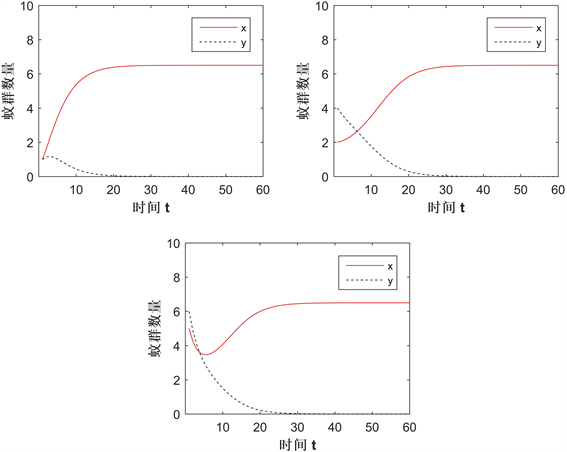Figure 2. The change in the solution of the system in Case A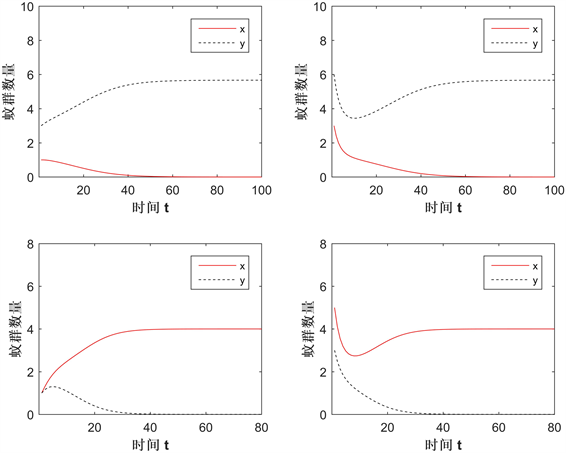Figure 3. The change in the solution of the system in Case BFigure 4. The change in the solution of the system in Case C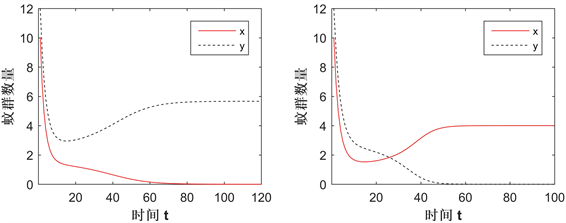Figure 5. The influence of CI strength changes in Case B

3. 总结

Modeling Wolbachia Propagation underIncomplete Cytoplasmic Incompatibility by Discrete Competition Model[J]. 应用数学进展, 2020, 09(02): 153-165. https://doi.org/10.12677/AAM.2020.92018

1. 1. Kyle, J.L. and Harris. E. (2008) Global Spread and Persistence of Dengue. Annual Review of Microbiology, 62, 71-92.https://doi.org/10.1146/annurev.micro.62.081307.163005

2. 2. Bardina, S.V., Bunduc, P., Tripathi, S., et al. (2017) Enhancement of Zika Virus Pathogenesis by Preexisting Antiflavivirus Immunity. Science, 356, 175-180. https://doi.org/10.1126/science.aal4365

3. 3. Yen, J.H. and Barr, A.R. (1971) New Hypothesis of the Cause of Cytoplasmic Incompatibility in Culex pipiens L. Nature, 232, 657-658. https://doi.org/10.1038/232657a0

4. 4. Xi, Z.Y., Khoo, C.C., Dobson, S.L., et al. (2005) Wolbachia Establishment and Invasion in an Aedes aegypti Laboratory Population. Science, 310, 326-328. https://doi.org/10.1126/science.1117607

5. 5. Yu, J.S. (2018) Modeling Mos-quitoes Population Suppression Based on Delay Differential Equations. SIAM Journal of Applied Mathematics, 78, 3168-3187. https://doi.org/10.1137/18M1204917

6. 6. Axford, J.K., Ross, P.A., Yeap, H.L., et al. (2016) Fitness of wAlbB Wolbachia Infection in Aedes aegypti: Parameter Estimates in an Outcrossed Background and Potential for Population Invasion. American Journal of Tropical Medicine and Hygiene, 94, 507-516. https://doi.org/10.4269/ajtmh.15-0608

7. 7. Farkas, J.Z. and Hinow, P. (2010) Structured and Unstructured Con-tinuous Models for Wolbachia Infections. Bulletin of Mathematical Biology, 72, 2067-2088. https://doi.org/10.1007/s11538-010-9528-1

8. 8. Zheng, B., Tang, M.X. and Yu, J.S. (2014) Modeling Wolbachia Spread in Mosquitoes through Delay Differential Equations. SIAM Journal of Applied Mathematics, 74, 734-770. https://doi.org/10.1137/13093354X

9. 9. Hu, L.C., Tang, M.X., Wu, Z.D., et al. (2019) The Threshold Infection Level for Wolbachia Invasion in Random Environments. Journal Differential Equations, 266, 4377-4393. https://doi.org/10.1016/j.jde.2018.09.035

10. 10. 邱俊雄, 郭文亮, 郑波. 不完全CI下的Wolbachia动力学行为[J]. 广州大学学报, 2016, 15(6): 34-38.

11. 11. Leslie, P.H. and Gower, J.C. (1958) The Properties of a Stochastic Model for Two Competing Species. Biometrika, 45, 316-330. https://doi.org/10.1093/biomet/45.3-4.316

12. 12. Liu, P.Z. and Elaydi, S.N. (2001) Discrete Competitive and Cooperative Models of Lotka-Volterra Type. Journal of Computational Analysis and Applications, 3, 53-73. https://doi.org/10.1023/A:1011539901001

13. 13. Elaydi, S.N. (1999) An Introduction to Difference Equations. 2nd Edition, Springer, New York. https://doi.org/10.1007/978-1-4757-3110-1

14. 14. Caswell, H. (2001) Matrix Population Models: Construction, Analysis and Interpretation. 2nd Edition, Sinauer Associates, Inc., Sunderland, MA.